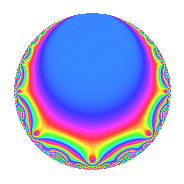# Properties

 Label 448.2.f.aLevel 448 Weight 2 Character orbit 448.f Analytic conductor 3.577 Analytic rank 1 Dimension 2 CM No Inner twists 2

# Related objects

## Newspace parameters

 Level: $$N$$ = $$448 = 2^{6} \cdot 7$$ Weight: $$k$$ = $$2$$ Character orbit: $$[\chi]$$ = 448.f (of order $$2$$ and degree $$1$$)

## Newform invariants

 Self dual: No Analytic conductor: $$3.57729801055$$ Analytic rank: $$1$$ Dimension: $$2$$ Coefficient field: $$\Q(\sqrt{-3})$$ Coefficient ring: $$\Z[a_1, \ldots, a_{7}]$$ Coefficient ring index: $$2$$ Sato-Tate group: $\mathrm{SU}(2)[C_{2}]$

## $q$-expansion

Coefficients of the $$q$$-expansion are expressed in terms of $$\beta = \sqrt{-3}$$. We also show the integral $$q$$-expansion of the trace form.

 $$f(q)$$ $$=$$ $$q -2 q^{3} -2 \beta q^{5} + ( -2 - \beta ) q^{7} + q^{9} +O(q^{10})$$ $$q -2 q^{3} -2 \beta q^{5} + ( -2 - \beta ) q^{7} + q^{9} + 2 \beta q^{11} + 2 \beta q^{13} + 4 \beta q^{15} -2 q^{19} + ( 4 + 2 \beta ) q^{21} + 2 \beta q^{23} -7 q^{25} + 4 q^{27} -6 q^{29} -8 q^{31} -4 \beta q^{33} + ( -6 + 4 \beta ) q^{35} + 2 q^{37} -4 \beta q^{39} + 4 \beta q^{41} -6 \beta q^{43} -2 \beta q^{45} + ( 1 + 4 \beta ) q^{49} -6 q^{53} + 12 q^{55} + 4 q^{57} -6 q^{59} + 2 \beta q^{61} + ( -2 - \beta ) q^{63} + 12 q^{65} + 2 \beta q^{67} -4 \beta q^{69} -2 \beta q^{71} -4 \beta q^{73} + 14 q^{75} + ( 6 - 4 \beta ) q^{77} -2 \beta q^{79} -11 q^{81} + 6 q^{83} + 12 q^{87} + 4 \beta q^{89} + ( 6 - 4 \beta ) q^{91} + 16 q^{93} + 4 \beta q^{95} -8 \beta q^{97} + 2 \beta q^{99} +O(q^{100})$$ $$\operatorname{Tr}(f)(q)$$ $$=$$ $$2q - 4q^{3} - 4q^{7} + 2q^{9} + O(q^{10})$$ $$2q - 4q^{3} - 4q^{7} + 2q^{9} - 4q^{19} + 8q^{21} - 14q^{25} + 8q^{27} - 12q^{29} - 16q^{31} - 12q^{35} + 4q^{37} + 2q^{49} - 12q^{53} + 24q^{55} + 8q^{57} - 12q^{59} - 4q^{63} + 24q^{65} + 28q^{75} + 12q^{77} - 22q^{81} + 12q^{83} + 24q^{87} + 12q^{91} + 32q^{93} + O(q^{100})$$

## Character Values

We give the values of $$\chi$$ on generators for $$\left(\mathbb{Z}/448\mathbb{Z}\right)^\times$$.

 $$n$$ $$127$$ $$129$$ $$197$$ $$\chi(n)$$ $$-1$$ $$-1$$ $$1$$

## Embeddings

For each embedding $$\iota_m$$ of the coefficient field, the values $$\iota_m(a_n)$$ are shown below.

For more information on an embedded modular form you can click on its label.

Label $$\iota_m(\nu)$$ $$a_{2}$$ $$a_{3}$$ $$a_{4}$$ $$a_{5}$$ $$a_{6}$$ $$a_{7}$$ $$a_{8}$$ $$a_{9}$$ $$a_{10}$$
447.1
 0.5 + 0.866025i 0.5 − 0.866025i
0 −2.00000 0 3.46410i 0 −2.00000 1.73205i 0 1.00000 0
447.2 0 −2.00000 0 3.46410i 0 −2.00000 + 1.73205i 0 1.00000 0
 $$n$$: e.g. 2-40 or 990-1000 Significant digits: Format: Complex embeddings Normalized embeddings Satake parameters Satake angles

## Inner twists

Char. orbit Parity Mult. Self Twist Proved
1.a Even 1 trivial yes
28.d Even 1 yes

## Hecke kernels

This newform can be constructed as the kernel of the linear operator $$T_{3} + 2$$ acting on $$S_{2}^{\mathrm{new}}(448, [\chi])$$.